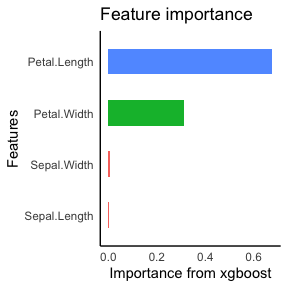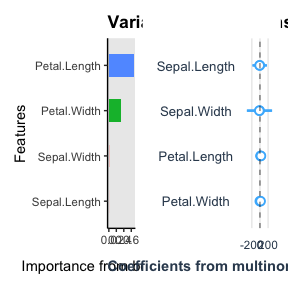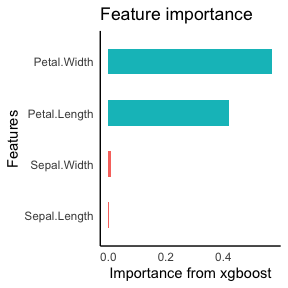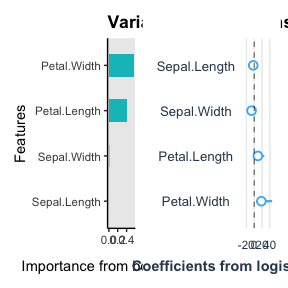# autostats

library(autostats)

## plot variable contributions

### multiclass target

Species is a 3-level factor so it will be automatically modelled with a multiclass neural network and a light gbm with multiclass objective function.

First set define the formula to use for modeling.

iris %>%
tidy_formula(target = Species) -> species_formula

species_formula
#> Species ~ Sepal.Length + Sepal.Width + Petal.Length + Petal.Width
#> <environment: 0x7fb691044d30>
iris %>%
auto_variable_contributions(species_formula)iris %>%
auto_model_accuracy(species_formula)
 4 - fold cross-validated accuracy for classification model of Species on dataset iris model metric mean_score std_err xgboost accuracy 0.960 0.01363 roc_auc 0.995 0.00406

### binary target

Linear models uses weighted logistic regression for modeling the coefficients

iris %>%
filter(Species != "setosa") %>%
auto_variable_contributions(species_formula)For the variable contributions the linear model uses penalized logistic regression provided by glmnet.

iris %>%
filter(Species != "setosa") -> iris_binary

iris_binary %>%
auto_model_accuracy(species_formula)
 4 - fold cross-validated accuracy for classification model of Species on dataset iris_binary model metric mean_score std_err xgboost accuracy 0.92 0.049 roc_auc 0.98 0.020

### continuous target

Models are automatically adapted for a continuous target.

Define the new formula

iris %>%
tidy_formula(target = Petal.Length) -> petal_formula

petal_formula
#> Petal.Length ~ Sepal.Length + Sepal.Width + Petal.Width + Species
#> <environment: 0x7fb67e3ea038>
iris %>%
auto_model_accuracy(petal_formula)
 4 - fold cross-validated accuracy for regression model of Petal.Length on dataset iris model metric mean_score std_err xgboost rmse 0.279 0.01256 rsq 0.974 0.00262

## auto anova

auto anova automatically regresses each continuous variable supplied against each categorical variable supplied. Lm is called separately for each continuous/ categorical variable pair, but the results are reported in one dataframe. Whether the outcome differs amongst categorical levels is determined by the p.value. The interpretation is affected by the choice of baseline for comparison. Traditionally the first level of the factor is used, however option to use the mean of the continuous variable as the baseline intercept is a helpful comparison.

iris %>%
auto_anova(Species, matches("Petal"), baseline = "first_level")
#> # A tibble: 6 × 12
#>   target   predictor level    estimate target_mean     n std.error level_p.value
#>   <chr>    <chr>     <chr>       <dbl>       <dbl> <int>     <dbl>         <dbl>
#> 1 Petal.L… Species   (Interc…    1.46        1.46     50    0.0609      9.30e-53
#> 2 Petal.L… Species   versico…    2.80        4.26     50    0.0861      5.25e-69
#> 3 Petal.L… Species   virgini…    4.09        5.55     50    0.0861      4.11e-91
#> 4 Petal.W… Species   (Interc…    0.246       0.246    50    0.0289      1.96e-14
#> 5 Petal.W… Species   versico…    1.08        1.33     50    0.0409      1.25e-57
#> 6 Petal.W… Species   virgini…    1.78        2.03     50    0.0409      7.95e-86
#> # … with 4 more variables: level_significance <chr>, predictor_p.value <dbl>,
#> #   predictor_significance <chr>, conclusion <chr>